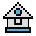Home

# GET Statement (Graphics)

#### Purpose:

To transfer graphics images from the screen.

#### Syntax:

`GET (x1,y1)-(x2,y2),array name`

The PUT and GET statements are used to transfer graphics images to and from the screen. PUT and GET make animation and high-speed object motion possible in either graphics mode.

The GET statement transfers the screen image bounded by the rectangle described by the specified points into the array. The rectangle is defined the same way as the rectangle drawn by the LINE statement using the ,B option.

The array is used simply as a place to hold the image, and can be of any type except string. It must be dimensioned large enough to hold the entire image. The contents of the array after a GET will be meaningless when interpreted directly (unless the array is of the type integer, as shown below).

The storage format in the array is as follows:

• 2 bytes given x dimension in bits
• 2 bytes given y dimension in bits
• the array data itself

The data for each row of pixels is left-justified on a byte boundary. If less than a multiple of eight bits is stored, the rest of the byte will be filled out with zeros. The required array size in bytes is as follows:

`4+INT((x*bitsperpixel+7)/8)*y`

See the SCREEN statement for bits-per-pixel values for different screen modes.

The bytes-per-element of an array are as follows:

• 2 for integer
• 4 for single-precision
• 8 for double-precision

The number of bytes required to get a 10 by 12 image into an integer array is 4+INT((10*2+7)/8)*12, or 40 bytes. An integer array with at least 20 elements is necessary.

If OPTION BASE equals zero, an integer array can be used to examine the x and y dimensions and the data. The x dimension is in element 0 of the array, and the y dimension is in element 1. Integers are stored low byte first, then high byte, but data is transferred high byte first (left-most), then low byte.

It is possible to get an image in one mode and put it in another, although the effect may be quite strange because of the way points are represented in each mode.

#### Examples:

```10 CLS: SCREEN 1
20 PSET(130, 120)
30 DRAW "U25; E7; R20; D32; L6; U12; L14"
40 DRAW "D12;L6":PSET(137,102)
50 DRAW "U4; E4; R8; D8; L12"
60 PSET(137, 88)
70 DRAW "E4; R20; D32; G4": PAINT(139, 87)
80 DIM A(500)
90 GET (125, 130)-(170, 80),A
100 FOR I=1 TO 1000: NEXT I
110 PUT (20,20), A, PSET
120 FOR I=1 TO 1000: NEXT I
130 GET (125,130)-(170, 80),A
140 FOR I=1 TO 1000: NEXT I
150 PUT (220,130), A, PRESET```